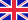•# Cartesian Coordinate System

Autor: Frederic P. Miller

High Quality Content by WIKIPEDIA articles! A Cartesian coordinate system specifies each point uniquely in a plane by a pair of numerical coordinates, which are the signed distances from the point to two fixed perpendicular directed lines, measured in the... Viac o knihe

Na objednávku, dodanie 2-4 týždne

60.35 €

bežná cena: 71.00 €

## O knihe

High Quality Content by WIKIPEDIA articles! A Cartesian coordinate system specifies each point uniquely in a plane by a pair of numerical coordinates, which are the signed distances from the point to two fixed perpendicular directed lines, measured in the same unit of length. Each reference line is called a coordinate axis or just axis of the system, and the point where they meet is its origin. The coordinates can also be defined as the positions of the perpendicular projections of the point onto the two axes, expressed as a signed distances from the origin. One can use the same principle to specify the position of any point in three-dimensional space by three Cartesian coordinates, its signed distances to three mutually perpendicular planes (or, equivalently, by its perpendicular projection onto three mutually perpendicular lines). In general, one can specify a point in spaces of any dimension n by use of n Cartesian coordinates, the signed distances from n mutually perpendicular hyperplanes. Cartesian coordinate system with the circle of radius 2 centered at the origin marked in red. The equation of the circle is x2 + y2 = 22.

• Vydavateľstvo: Alphascript Publishing
• Rok vydania: 2009
• Formát: Paperback
• Rozmer: 220 x 150 mm
• Jazyk: Anglický jazyk
• ISBN: 9786130245283

Generuje redakčný systém BUXUS CMS spoločnosti ui42.